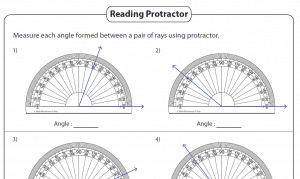# Angles: Printable Worksheets (4th - 7th Grade)Angles Worksheets - Angle worksheets cover almost all aspects of angle topics in geometry. It contains naming angles in different ways, identifying parts of the angles, classifying types, measuring angles with protractor, complementary and supplementary angles, angles formed between intersecting lines, simple algebra problems based on angles, angles formed by parallel lines and a transversal and more. It also contains angle worksheets based on geometric shapes such as triangle, quadrilateral, polygon and more.

Draw and identify lines and angles, and classify shapes by properties of their lines and angles.

CCSS.Math.Content.4.G.A.1 - Draw points, lines, line segments, rays, angles (right, acute, obtuse), and perpendicular and parallel lines. Identify these in two-dimensional figures.
CCSS.Math.Content.4.G.A.2 - Classify two-dimensional figures based on the presence or absence of parallel or perpendicular lines, or the presence or absence of angles of a specified size. Recognize right triangles as a category, and identify right triangles.

Geometric measurement: understand concepts of angle and measure angles.

CCSS.Math.Content.4.MD.C.5 - Recognize angles as geometric shapes that are formed wherever two rays share a common endpoint, and understand concepts of angle measurement:
CCSS.Math.Content.4.MD.C.5.a  - An angle is measured with reference to a circle with its center at the common endpoint of the rays, by considering the fraction of the circular arc between the points where the two rays intersect the circle. An angle that turns through 1/360 of a circle is called a "one-degree angle," and can be used to measure angles.
CCSS.Math.Content.4.MD.C.5.b - An angle that turns through n one-degree angles is said to have an angle measure of n degrees.
CCSS.Math.Content.4.MD.C.6  - Measure angles in whole-number degrees using a protractor. Sketch angles of specified measure.
CCSS.Math.Content.4.MD.C.7  - Recognize angle measure as additive. When an angle is decomposed into non-overlapping parts, the angle measure of the whole is the sum of the angle measures of the parts. Solve addition and subtraction problems to find unknown angles on a diagram in real world and mathematical problems, e.g., by using an equation with a symbol for the unknown angle measure.
CCSS.MATH.CONTENT.7.G.B.5
Use facts about supplementary, complementary, vertical, and adjacent angles in a multi-step problem to write and solve simple equations for an unknown angle in a figure.